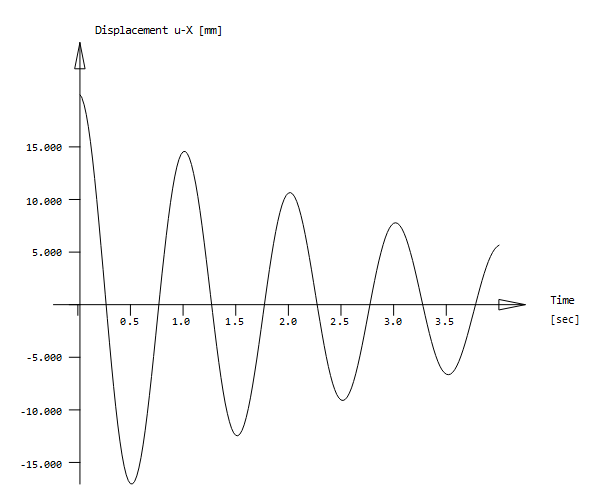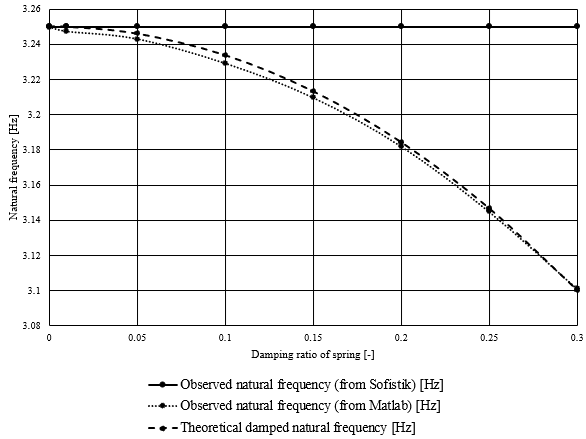# Cannot simulate effect of damping in SDOF model on natural frequency

Hello,

I’ve modeled a SDOF system in SOFiSTiK with Teddy in which I played around with the three variables of mass ‘m’, spring constant ‘k’ and damping coefficient ‘c’ and observed the effect on the natural frequency of the system. My objective was to compare the resulting natural frequency to a theoretically calculated one, and if all goes well they should be (almost) the same.Based on the theoretical equations (that can for example be found at the URL below), I derived the following expression for the damped angular natural frequency w_d:

omega_d = sqrt(k/m - c²/(4m²))

Dividing this by 2*pi then gives the natural frequency in Hz, which can be compared to the values given by SOFiSTiK.

This seems to work fine when the damping coefficient is 0, which means I could change the mass and spring constant in any way possible, and the observed and theoretical natural frequencies were always the same. The problem is that the damping coefficient doesn’t seem to have any effect on the natural frequency in the SOFiSTiK model, which I don’t quite understand. Even if I change it to excessively large values, there is no effect on the natural frequency whatsoever.

The SOFIMSHA script to create the model is shown below. I’ve also attached my entire Teddy file to this post.

NODE NO 1 X 0 Y 0 Z 0 FIX F
NODE NO 2 X 0 Y 0 Z #spring_height FIX ZP
NODE NO 3 X 0 Y 0 Z #spring_height+#sdof_height FIX ZP

TRUS NO 1 NA 2 NE 3 NCS #sdof_cs
SPRI NO 1 NA 1 NE 2 DZ 1 CP #spring_cst DP #damping

SDOF v1.dat (6.2 KB)

Any help will be greatly appreciated. Thank you for reading.

Kind regards

Hello

On our website we have a verification example about an undercritically damped system.

www.sofistik.com > Infocenter - Overview > Documentation - Tutorials > Verification Benchmarks > Mechanical Benchamrks > BE24 - Free Vibration of a Under-critically Damped SDOF System

If you add a new +PROG DYNA calculation with the command “EIGE” to the end of the example, you should see the consequences of a damped spring element.

• As expected, the circular eigenfrequency ω varies according to the damping ξ

Best regards
Frederik Höller

Thank you for your response, mr. Höller. I’m not sure if I fully understand though, I examined the example you referred to and I tried to re-calculate the natural frequency by adding the following code at the end:

+prog dyna urs:15
grp -
eige 1 lc 9001
end

However, the natural frequency is still just 1.00 Hz, even if I increase the damping coefficient (DP) of the spring element to a very high number, e.g. 1000.

Is the sentence “As expected, the circular eigenfrequency varies according to the damping” quoted from somewhere, if I may ask? I’m asking because I didn’t see it anywhere in the PDF of the benchmark example no. 24.

Kind regards

Ok no I see the problem.
SOFiSTiK calculates and displays the undamped eigenvalue and circular eigenfrequency ω. The damped circular eigenfrequency can be calculated manually with the formula ω_d = sqrt(1-xi²).

But the damping is taken into account for further calculations. As the example BE24 shows:

FEDE 1 1 2 CP 4*#pi^2 dp 2*#pi/10
-> Xi = 5 %FEDE 1 1 2 CP 4*#pi^2 dp 10*#pi/10
-> Xi = 25 %

Best regards

Thank you again, mr. Höller! Based on what you said, I did some tests and it is indeed taken into account for further calculations.

I did several simulations with different damping coefficients of the spring (which are related to the damping ratios as shown on the graph below) and then determined the natural frequency based on the resulting acceleration data with a Matlab script. The observed natural frequency is very close to the theoretical damped natural frequency calculated from the formula.Kind regards

1 Like